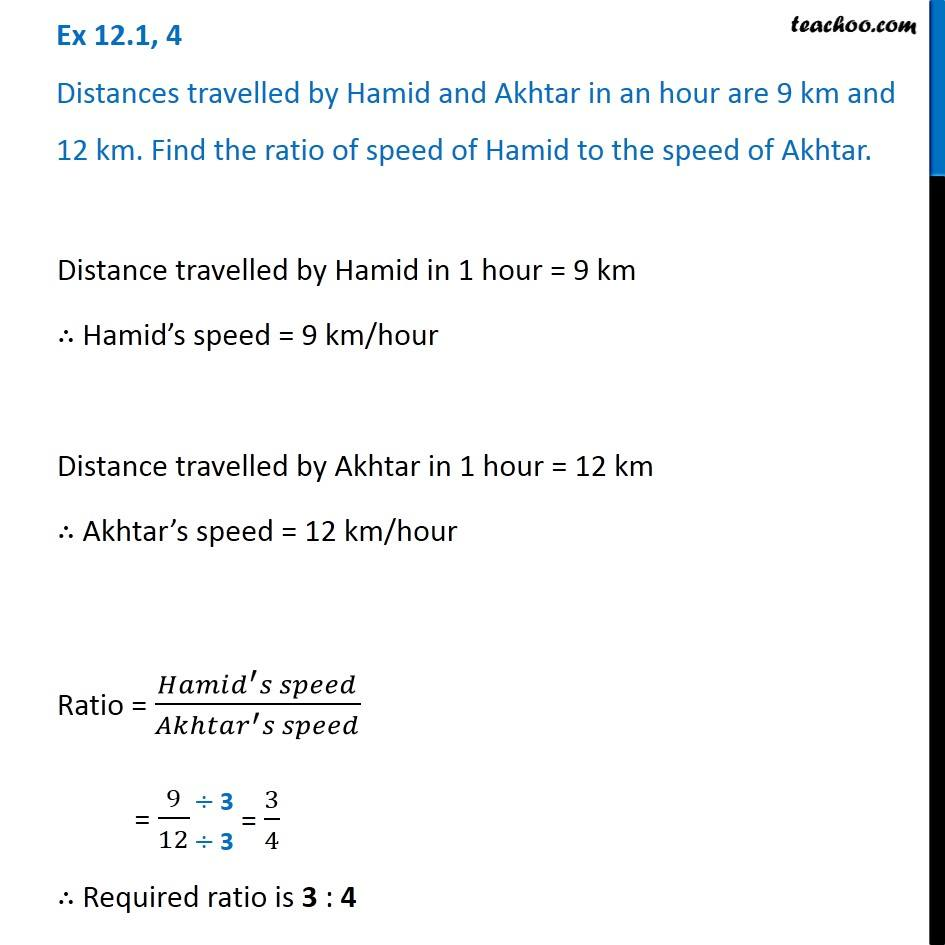Ex 12.1

Chapter 12 Class 6 Ratio And Proportion
Serial order wiseGet live Maths 1-on-1 Classs - Class 6 to 12

### Transcript

Ex 12.1, 4 Distances travelled by Hamid and Akhtar in an hour are 9 km and 12 km. Find the ratio of speed of Hamid to the speed of Akhtar. Distance travelled by Hamid in 1 hour = 9 km ∴ Hamid’s speed = 9 km/hour Distance travelled by Akhtar in 1 hour = 12 km ∴ Akhtar’s speed = 12 km/hour Ratio = (𝐻𝑎𝑚𝑖𝑑^′ 𝑠 𝑠𝑝𝑒𝑒𝑑)/(𝐴𝑘ℎ𝑡𝑎𝑟^′ 𝑠 𝑠𝑝𝑒𝑒𝑑) = 9/12 ∴ Required ratio is 3 : 4